How Cheenta works to ensure student success?
Explore the Back-Story

# NMTC 2017 Stage II - GAUSS (Class 5, 6) - Problems and Solutions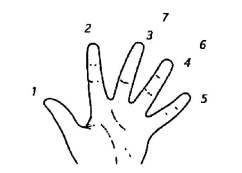###### Problem 1

If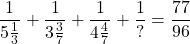Find what should be filled in the place marked?

###### Problem 2

There are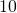cards numberedto. There are three second standard children Ram, Bilal and Cynthia. The teacher selects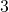cards from thecards without seeing the numbers. She distributes the cards to the children one to each. After the children noting down the numbers in the cards she collects them back. Again she repeats the same process two more times. So, each child now hasnumbers noted down. The teacher asks them to add the numbers and tell her the sums obtained by them. They told her that the sums were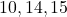But Ram received the same cards three times. Bilal and Cynthia received all cards different. What numbered cards are received by each? Write down the steps you used to get the answer.

###### Problem 3

In the adjoining figure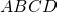is a rectangle. Points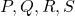are marked as in the diagram such that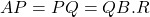is the midpoint of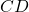. If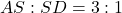, find the ratio of the areas of triangle, quadrilateral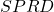, triangleand the trapezium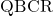.

###### Problem 4

Take the numbers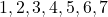and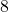. We have to make two groups,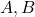each containing four numbers such that
(a) The sum of the numbers in groupis equal to the sum of the numbers in group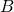(b) Grouphas a number such that when it is moved from group A to group B, the sum of the five numbers in groupis equal to twice the sum of the 3 numbers in group.
(c) Grouphas a number such that when it moved to group, the sum of the three numbers in Group B isof the sum of the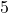numbers in Group A.

Find the number in the groups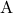and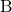.

###### Problem 5

Mahadevan was puzzled by the strange way in which his grand daughter was counting. She began to count on the fingers of her left hand. She started by calling the thumb 1 , the first finger 2 , middle finger 3 , ring finger 4 , little finger 5 , then she reversed direction, calling the ring finger 6 , middle finger 7 , first finger 8 , thumb 9 , then back to the first finger for 10 , middle finger for 11 , and so on. She continued to count back and forth in this peculiar manner until she reached a count of 20 on her ring finger.

Seeing this, Mahadevan told her "If you can find on which finger you will count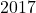, I will buy you an ice cream". Can you find on which finger she will count? Explain the steps you used to arrive at the answer.

###### Problem 6

In the adjoining figure the number in each circle is the sum of the numbers in the two adjacent circles below it.

(a)Find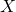, writing the steps systematically.
(b)What is the least positive number to be added toso that the result is a perfect square?
(c)What is the least positive number to be subtracted fromso that the result is a perfect square?

###### Problem 1

IfFind what should be filled in the place marked?

###### Problem 2

There arecards numberedto. There are three second standard children Ram, Bilal and Cynthia. The teacher selectscards from thecards without seeing the numbers. She distributes the cards to the children one to each. After the children noting down the numbers in the cards she collects them back. Again she repeats the same process two more times. So, each child now hasnumbers noted down. The teacher asks them to add the numbers and tell her the sums obtained by them. They told her that the sums wereBut Ram received the same cards three times. Bilal and Cynthia received all cards different. What numbered cards are received by each? Write down the steps you used to get the answer.

###### Problem 3

In the adjoining figureis a rectangle. Pointsare marked as in the diagram such thatis the midpoint of. If, find the ratio of the areas of triangle, quadrilateral, triangleand the trapezium.

###### Problem 4

Take the numbersand. We have to make two groups,each containing four numbers such that
(a) The sum of the numbers in groupis equal to the sum of the numbers in group(b) Grouphas a number such that when it is moved from group A to group B, the sum of the five numbers in groupis equal to twice the sum of the 3 numbers in group.
(c) Grouphas a number such that when it moved to group, the sum of the three numbers in Group B isof the sum of thenumbers in Group A.

Find the number in the groupsand.

###### Problem 5

Mahadevan was puzzled by the strange way in which his grand daughter was counting. She began to count on the fingers of her left hand. She started by calling the thumb 1 , the first finger 2 , middle finger 3 , ring finger 4 , little finger 5 , then she reversed direction, calling the ring finger 6 , middle finger 7 , first finger 8 , thumb 9 , then back to the first finger for 10 , middle finger for 11 , and so on. She continued to count back and forth in this peculiar manner until she reached a count of 20 on her ring finger.

Seeing this, Mahadevan told her "If you can find on which finger you will count, I will buy you an ice cream". Can you find on which finger she will count? Explain the steps you used to arrive at the answer.

###### Problem 6

In the adjoining figure the number in each circle is the sum of the numbers in the two adjacent circles below it.

(a)Find, writing the steps systematically.
(b)What is the least positive number to be added toso that the result is a perfect square?
(c)What is the least positive number to be subtracted fromso that the result is a perfect square?

This site uses Akismet to reduce spam. Learn how your comment data is processed.

### Knowledge Partner